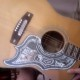### old mole-skin sketch newly inkedhere one has labeled the vertices of a cube
with (“euclidean”) 3-space co-ordinates.
the “origin”… we can think of it as
our “point of view”… is at 000.

[the “point” in 3-space usually
denoted (x, y, z) is here written
as “xyz”; we restrict our attention
to the values in {0, 1} for these
variables (since the (8) points
000, 001, 010, 011,
100, 101, 110, 111
then form the vertices of our cube).]

putting the primary colors (Red, Yellow, & Blue)
“on the x, y, & z axes (respectively)”, i.e., putting
Red—>001
Yel—>010
Blu—>100,
we may then conveniently put the *secondaries*
(Green, Orange, & Purple) at the “third vertex” of
the back left wall (“G = Y + B”),
the front left wall (“P = R + B”), and
the floor (“O = R + Y”)
(respectively).

the last vertex is the “ideal” point 111;
all the colors blend here to form Mud.

cool algebra ensues. but not till after dinner.
or you could look it up.

1.vlorbik